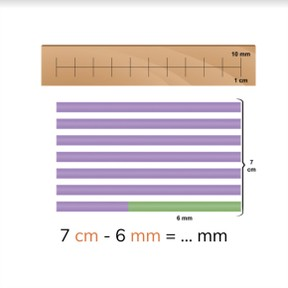Subtracting mm and cm

# Subtracting mm and cm

Students learn to subtract millimeters and centimeters.

No account needed.8,000 schools use Gynzy92,000 teachers use Gynzy1,600,000 students use Gynzy

## General

Students learn how they can subtract millimeters and centimeters from each other.

## Standards

CCSS.Math.Content.4.MD.A.2

## Learning objective

Students will be able to subtract millimeters (mm) and centimeters (cm) from each other.

## Introduction

Show the images on the interactive whiteboard. With each image is a measurement that the students must convert.

## Instruction

Ask the students how many millimeters are in one centimeter. Show with help from a visual aid how you can subtract centimeters and millimeters from each other. State that you must first convert centimeters to millimeters, then you can subtract them from each other. Have the students also practice on their own with help from a visual aid. Then you explain that you can subtract centimeters and millimeters from each other, by first converting the centimeters to millimeters. To do this you take one jump to the right, so you multiply by 10. Discuss a number of problems together with the class. Next the students must solve a subtraction problem, for which they can either choose from a few possible solutions or give the answer by themselves. Sometimes you don't have a round number left over, but you also have a few millimeters left over. In that case, see how many centimeters you have and then how many millimeters you have. Discuss several problems with the class, for which you must first convert the centimeters to millimeters and then you can solve the problem. These problems ask for the number of centimeters and millimeters that you have. The answer that you have calculated in millimeters must then be divided by 10, to know how many centimeters and how many millimeters it is. Next you discuss subtracting centimeters and millimeters by means of a story problem. Have the students solve a story problem on their own.

To check whether students can subtract millimeters and centimeters from each other, you can ask the following questions:
- How do you subtract centimeters and millimeters from each other, when you must give the answer in millimeters?
- How do you subtract centimeters and millimeters from each other, when you must give the answer in centimeters?

## Quiz

Students first practice subtracting millimeters and centimeters, for which they get a visual aid. Then they practice subtracting, where they are only given the numbers and then they make a story problem, which they must solve.

## Closing

You discuss with the students again that it is important to be able to subtract with millimeters and centimeters, because that is how you can determine how much you have left of something. Check if they know that they must first convert to the same length measurement by multiplying or dividing by 10. Have the students practice in pairs subtracting millimeters and centimeters. The students write measurements in centimeters and millimeters on cards and make piles of each measurement. They take turns taking one card from each pile, they say the problem and solve it.

## Teaching tips

When students have difficulty with subtracting millimeters and centimeters, you can give them a diagram of the metric system, on which you only have millimeters and centimeters with an arrow for ÷ 10 and an arrow for x 10. Explain that you must first convert. Then you can subtract the numbers from each other.

## Instruction materials

You may print out a diagram of the metric system.

### The online teaching platform for interactive whiteboards and displays in schools

• Save time building lessons

• Manage the classroom more efficiently

• Increase student engagement